## สินค้าขายดีล่าสุดระหว่างวันที่ 01/04/2023 ถึง 01/04/2023

ลำดับ  สินค้าราคา(บาท)
18858722002633
Based on the Basic Education Curriculum B.E. 2551 (Revised Edition B.E. 2560)
145.00
2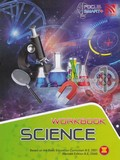8858722002398
Based on the Basic Education Curriculum B.E. 2551 (Revised Edition B.E. 2560)
145.00
3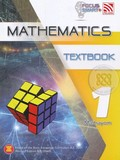9786165413060
Based on the Basic Education Curriculum B.E. 2551 (Revised Edition B.E. 2560)
285.00
4160.00
5160.00
6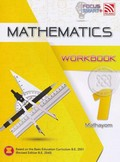8858722002404
Based on the Basic Education Curriculum B.E. 2551 (Revised Edition B.E. 2560)
145.00
7160.00
8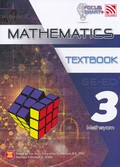9786165413145
Based on the Basic Education Curriculum B.E. 2551 (Revised Edition B.E. 2560)
285.00
9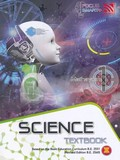9786165413053
Based on the Basic Education Curriculum B.E. 2551 (Revised Edition B.E. 2560)
285.00
10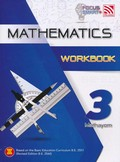8858722002664
Based on the Basic Education Curriculum B.E. 2551 (Revised Edition B.E. 2560)
145.00
11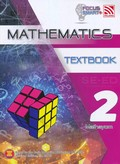9786165413138
Based on the Basic Education Curriculum B.E. 2551 (Revised Edition B.E. 2560)
285.00
12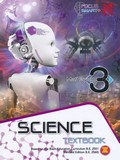9786165413169
Based on the Basic Education Curriculum B.E. 2551 (Revised Edition B.E. 2560)
285.00
13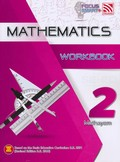8858722002657
Based on the Basic Education Curriculum B.E. 2551 (Revised Edition B.E. 2560)
145.00
14160.00
159786165413152
Based on the Basic Education Curriculum B.E. 2551 (Revised Edition B.E. 2560)
285.00
16160.00
17160.00
18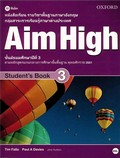215.00
19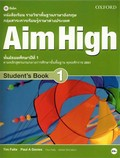215.00
20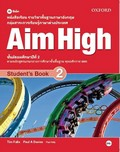215.00
12345